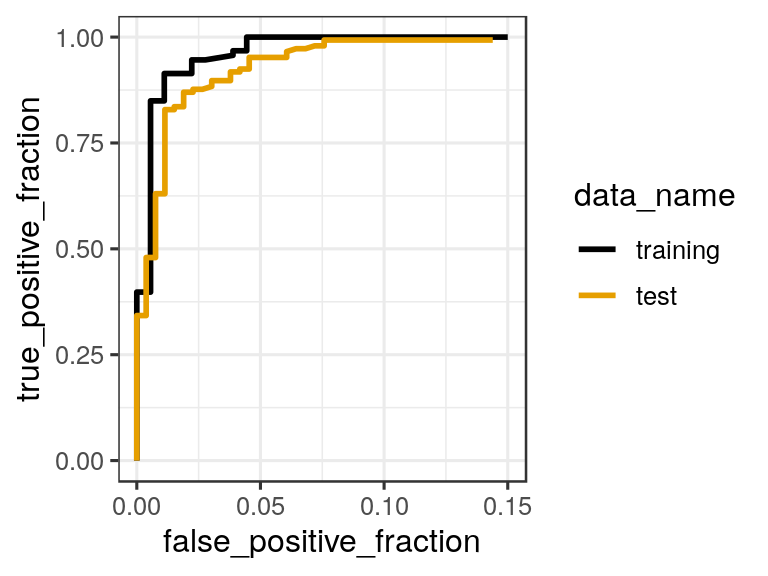## In-class worksheet 13

March 3, 2020

In this worksheet, we will use the libraries tidyverse, plotROC, and ggthemes:

library(tidyverse)
theme_set(theme_bw(base_size=12)) # set default ggplot2 theme
library(plotROC)
library(ggthemes)

# 1. Working with training and test data sets

We continue working with the biopsy data set:

biopsy <- read_csv("http://wilkelab.org/classes/SDS348/data_sets/biopsy.csv")
## Parsed with column specification:
## cols(
##   clump_thickness = col_double(),
##   uniform_cell_size = col_double(),
##   uniform_cell_shape = col_double(),
##   epithelial_cell_size = col_double(),
##   bare_nuclei = col_double(),
##   bland_chromatin = col_double(),
##   normal_nucleoli = col_double(),
##   mitoses = col_double(),
##   outcome = col_character()
## )
biopsy$outcome <- factor(biopsy$outcome) # make outcome a factor

The following code splits the biopsy data set into a random training and test set:

train_fraction <- 0.4 # fraction of data for training purposes
set.seed(126)  # set the seed to make the partition reproductible
train_size <- floor(train_fraction * nrow(biopsy)) # number of observations in training set
train_indices <- sample(1:nrow(biopsy), size = train_size)

train_data <- biopsy[train_indices, ] # get training data
test_data <- biopsy[-train_indices, ] # get test data

Fit a logistic regression model on the training data set, then predict the outcome on the test data set, and plot the resulting ROC curves. Limit the x-axis range from 0 to 0.15 to zoom into the ROC curve. (Hint: Do not use coord_fixed().)

# model to use:
# outcome ~ clump_thickness + uniform_cell_size + uniform_cell_shape

glm_out <- glm(
outcome ~ clump_thickness + uniform_cell_size + uniform_cell_shape,
data = train_data,
family = binomial
)

# results data frame for training data
df_train <- data.frame(
predictor = predict(glm_out, train_data),
known_truth = train_data$outcome, data_name = "training" ) # results data frame for test data df_test <- data.frame( predictor = predict(glm_out, test_data), known_truth = test_data$outcome,
data_name = "test"
)

df_combined <- rbind(df_train, df_test)

ggplot(df_combined, aes(d = known_truth, m = predictor, color = data_name)) +
geom_roc(n.cuts = 0) +
xlim(0, 0.15) +
scale_color_colorblind()
## Warning in verify_d(datad): D not labeled 0/1, assuming benign = 0 and ## malignant = 1!# 2. Area under the ROC curves You can calculate the areas under the ROC curves by running calc_auc() on a plot generated with geom_roc() (see previous worksheet). Use this function to calculate the area under the training and test curve for the model outcome ~ clump_thickness. For this exercise, generate a new set of training and test datasets with a different fraction of training data from before. train_fraction <- 0.2 # fraction of data for training purposes set.seed(123) # set the seed to make the partition reproductible train_size <- floor(train_fraction * nrow(biopsy)) # number of observations in training set train_indices <- sample(1:nrow(biopsy), size = train_size) train_data <- biopsy[train_indices, ] # get training data test_data <- biopsy[-train_indices, ] # get test data # fit the model on the training data glm_out <- glm( outcome ~ clump_thickness, data = train_data, family = binomial ) # predict outcomes for the training data df_train <- data.frame( predictor = predict(glm_out, train_data), known_truth = train_dataoutcome,
data_name = "training"
)

# predict outcomes for the test data
df_test <- data.frame(
predictor = predict(glm_out, test_data),
known_truth = test_data$outcome, data_name = "test" ) df_combined <- rbind(df_train, df_test) p <- ggplot(df_combined, aes(d = known_truth, m = predictor, color = data_name)) + geom_roc(n.cuts = 0) calc_auc(p) ## Warning in verify_d(data$d): D not labeled 0/1, assuming benign = 0 and
## malignant = 1!
##   PANEL group       AUC
## 1     1     1 0.9214427
## 2     1     2 0.9050554

# 3. If this was easy

Write code that combines the AUC values calculated by calc_auc() with the correct group names and orders the output in descending order of AUC. (Hint: We have seen similar code in the previous worksheet.)

data_name <- unique(df_combined$data_name) data_info <- data.frame( data_name, group = order(data_name) ) left_join(data_info, calc_auc(p)) %>% select(-group, -PANEL) %>% arrange(desc(AUC)) ## Warning in verify_d(data$d): D not labeled 0/1, assuming benign = 0 and
## malignant = 1!
## Joining, by = "group"
##   data_name       AUC
## 1  training 0.9214427
## 2      test 0.9050554